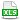HomeTechniques and Tips@RISK: General QuestionsGeometric Mean in @RISK

# 2.25. Geometric Mean in @RISK

Applies to:
@RISK, all releases

Question:
How can I obtain the geometric mean of an output in @RISK?

Response:

Unfortunately, @RISK doesn't have a function to get the geometric mean from an output directly but it is possible to compute it using the arithmetic mean of logarithms; it can be expressed as the exponential of the arithmetic mean of logarithms as described in the model attached.

last edited: 2019-04-16

•@RISK-Geometric-mean.xlsx